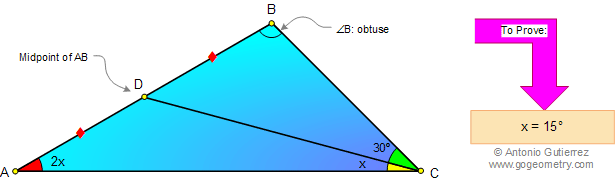# Geometry Problem 46 with Solution: Angles, Triangle, Midpoint, Double Angle, 15 Degrees

< PREVIOUS PROBLEM  |  NEXT PROBLEM >

The figure shows an obtuse triangle ABC (angle B is the obtuse angle), D is the midpoint of AB, the measure of angle BCD is 30 degrees and the measure of angle BAC is double the measure of angle ACD. Prove that the measure of angle ACD is 15°. .Home | Geometry | Problems | Open Problems | 10 Problems | 41-50 | Obtuse Triangle | Email | By Antonio Gutierrez Post or view a solution to the problem 46